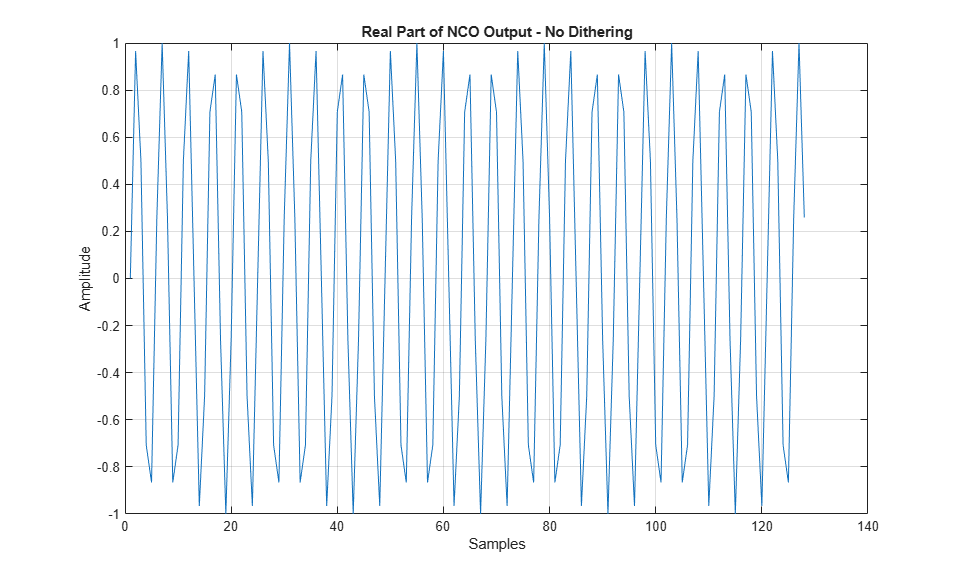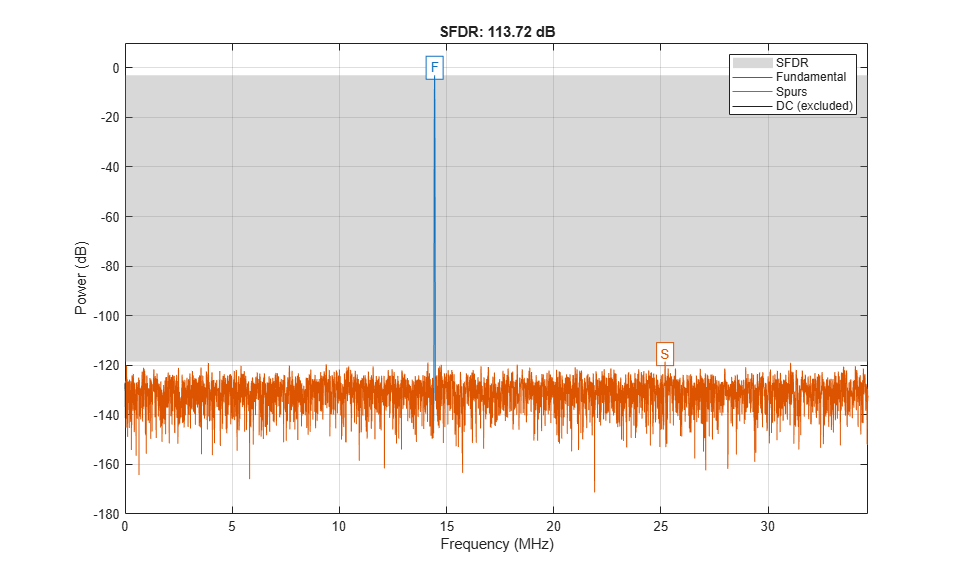Documentation

### This is machine translation

Mouseover text to see original. Click the button below to return to the English version of the page.

## Spurious-Free Dynamic Range (SFDR) Measurement

This example shows how to analyze a numerically controlled oscillator (NCO) of a digital down-converter (DDC) implemented in fixed-point arithmetic. The example measures the spurious free dynamic range (SFDR) of the NCO, and explore the effects of adding phase dither. The number of dither bits affects hardware implementation choices. The example shows trade-offs among noise floor level, spurious effects, and number of dither bits. The DDC in the example, designed to meet the GSM specification, models the Graychip 4016.

### Introduction

Numerically controlled oscillators (NCOs) are an efficient means of generating sinusoidal signals, and are useful when you require a continuous-phase sinusoidal signal with variable frequency.

A DDC is a key component of digital radios. It translates the high-input sample rates of a digital radio down to lower sample rates (baseband) for further and easier processing. Our DDC has an input rate of 69.333 MHz and is tasked with converting the rate down to 270.833 KHz in accordance with GSM specifications.

The DDC consists of an NCO and a mixer to quadrature down convert the input signal to baseband. A Cascaded Integrator-Comb (CIC) then low-pass filters the baseband signal, and along with two FIR decimating filters downsample the signal to achieve the desired low sample rate, which is then ready for further processing. The final stage, depending on the application, often includes a resampler that interpolates or decimates the signal to achieve the desired sample rate. Further filtering can be achieved with the resampler. See the block diagram of a typical DDC, below. Note that Simulink® handles complex signals, so we don't have to treat the I and Q channels separately.While this example focuses on the analysis of the NCO, an example titled "Implementing the Filter Chain of a Digital Down-Converter", focusing on designing the three-stage, multirate, fixed-point filter chain and HDL code generation is available in the DSP System Toolbox™.

### The Numerically Controlled Oscillator

The digital mixer section of the DDC includes a multiplier and an NCO, which provide channel selection or tuning for the radio. The mixer is basically a sine-cosine generator, creating complex values for each sine-cosine pair. The typical NCO has four components: the phase accumulator, the phase adder, the dither generator, and sine-cosine lookup table.

Here is a typical NCO circuit modeled in Simulink, similar to what you might see in the Graychip data sheet.

```open_system('ddcncomodel') ```Based on the input frequency, the NCO's phase accumulator produces values that address a sine-cosine lookup table. The phase adder specifies a phase offset that modulates the output of the phase accumulator. The Dither Generator provides phase dithering to reduce amplitude quantization noise, and improving the SFDR of the NCO. The Sine/Cosine Lookup block produces the actual complex sinusoidal signal, and the output is stored in the variable nco_nodither.

In the Graychip, the tuning frequency is specified as a normalized value relative to the chip's clock rate. So for a tuning frequency of F, the normalized frequency is F/Fclk, where Fclk is the chip's clock rate. The phase offset is specified in radians, ranging from 0 to 2pi. In this example the normalized tuning frequency is set to 5/24 while the phase offset is set to 0. The tuning frequency is specified as a 32-bit word and the phase offset is specified as a 16-bit word.

Since the NCO is implemented using fixed-point arithmetic, it experiences undesirable amplitude quantization effects. These numerical distortions are due to the effects of finite word length. Basically, sinusoids are quantized creating cumulative, deterministic, and periodic errors in the time domain. These errors, appear as line spectra or spurs in the frequency domain. The amount of attenuation from the peak of the signal of interest to the highest spur is the SFDR.

The SFDR of the Graychip is 106 dB, but the GSM specification requires that the SFDR be greater than 110 dB. There are several ways to improve the SFDR, and you will explore adding phase dither to the NCO.

The Graychip's NCO contains a phase dither generator which is basically a random integer generator used to improve the oscillator's output purity. Dithering causes the unintended periodicities of the quantization noise (which causes "spikes" in spectra and thus poor SFDR) to be spread across a broad spectrum, effectively reducing these undesired spectral peaks. Conservation of energy applies, however, so the spreading effectively raises the overall noise floor. That is, the dithering is good for SFDR, but only up to a point.

Let's run the NCO model and analyze its output in the MATLAB workspace. This model does not use dithering.

```sim('ddcncomodel') whos nco* ```
``` Name Size Bytes Class Attributes nco_nodither 1x1x20545 328720 double complex ```

The plot below displays the real part of the first 128 samples of the NCO's output, stored in the variable, nco_nodither.

```plot(real(squeeze(nco_nodither(1:128)))) grid title('Real Part of NCO Output - No Dithering') ylabel('Amplitude') xlabel('Samples') ```### SFDR of NCO Output

Let's take a look at the SFDR of the output of the NCO.

Calculate and plot the SFDR of the NCO output

```Fs = 69.333e6; sfdr(real(nco_nodither),Fs); ```As expected, the power spectrum plot shows a peak at 14.44 MHz, which is the NCO's tuning frequency, 5/24*Fs = 14.444 MHz.

The SFDR, however, is about 106.17 dB, which is too high to meet the GSM specification, which requires 110 dB or more. We can improve this dynamic range by use of phase dithering.

### Exploring the Effects of Dithering

To explore adding dither to the NCO, the NCO circuit shown above has been encapsulated in a subsystem and duplicated three times. A different amount of dither was selected for each NCO. Although the NCO allows a range of 1 to 19 bits of dither to be specified, you will try just few values. Running this model will produce three different NCO outputs based on the amount of dither added.

```open_system('ddcncowithdithermodel') ```Running the simulation will produce three signals in the MATLAB workspace that you can then analyze using the spectral analysis algorithm defined above. You can run the simulation from the model or from the command line using the sim command.

```sim('ddcncowithdithermodel') ```

After the simulation completes you are left with the signals that are the NCOs' output. Each signal shows a different amount of dithering.

```whos nco* ```
``` Name Size Bytes Class Attributes nco_dither3 1x1x20501 328016 double complex nco_dither5 1x1x20501 328016 double complex nco_dither7 1x1x20501 328016 double complex nco_nodither 1x1x20545 328720 double complex ```

Compute and plot the SFDR after adding 3 bits of dithering.

```figure sfdr(real(nco_dither3),Fs) ```
```ans = 107.6285 ```With three bits of dither added, the highest spur is now about -112 dB. The SFDR is 107.63 dB. It still fails to meet the GSM specification.

Now add 5 bits of dithering.

```figure sfdr(real(nco_dither5),Fs) ```
```ans = 123.4065 ```With five bits of dither added, the highest spur is now about -127 dB.

The SFDR is 123.41 dB, exceeding the GSM specification.

It appears that more dither gives better results, so add 7 bits of dithering.

```figure sfdr(real(nco_dither7),Fs) ```
```ans = 113.7189 ```Note that our computed SFDR degraded to 113.72 dB. This is due to the broadband noise generated by dithering starting to overtake the power of the spurious content.

Using 7 bits of dithering meets the GSM specification, but is not as effective as using 5 bits of dithering.

### Comparing Results

Tabulate the SFDR for each NCO output against the amounts of dithering for each NCO output.

```Number of Spur Free Dynamic Dither bits Range(dB)```
` 0 106.17`
` 3 107.63`
` 5 123.41`
` 7 113.72`

### Summary

This example analyzed the output of an NCO used in a digital down converter for a GSM application. Spectral analysis was used to measure the SFDR, the difference between the highest spur and the peak of the signal of interest. Spurs are deterministic, periodic errors that result from quantization effects. The example also explored the effects of adding dither in the NCO, which adds random data to the NCO to improve its purity. We found that using five bits of dithering achieved the highest SFDR.